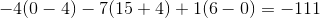Calculus 3 : Vectors and Vector Operations

Example Questions

Example Question #741 : Vectors And Vector Operations

Find the determinant of the 3x3 matrix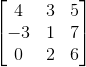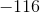Explanation:

To find the determinant of a 3x3 matrix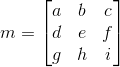, we use the formula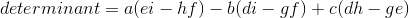Using the matrix from the problem statement and applying, we get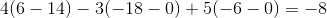Example Question #742 : Vectors And Vector Operations

Find the determinant of the 3x3 matrix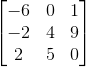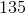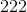Explanation:

To find the determinant of a 3x3 matrix, we use the formulaUsing the matrix from the problem statement and applying, we get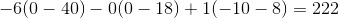Example Question #743 : Vectors And Vector Operations

Find the determinant of the 2x2 matrix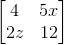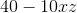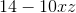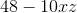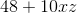Explanation:

To find the determinant of a 2x2 matrix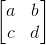we use the definition: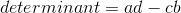Applying to the matrix from the problem statement, we get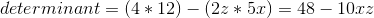Example Question #744 : Vectors And Vector Operations

Find the determinant of the 2x2 matrix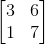Explanation:

To find the determinant of a 2x2 matrix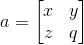, we apply the definition: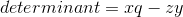Using the matrix from the problem statement, we get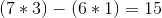Example Question #745 : Vectors And Vector Operations

Find the determinant of the 2x2 matrix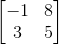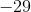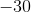Explanation:

To find the determinant of a 2x2 matrix, we apply the definition:Using the matrix from the problem statement, we get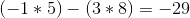Example Question #746 : Vectors And Vector Operations

Find the determinant of the 2x2 matrix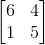Explanation:

To find the determinant of a 2x2 matrix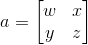, we use the formula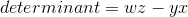Applying to the matrix from the problem statement, we get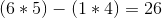Example Question #747 : Vectors And Vector Operations

Find the determinant of the 2x2 matrix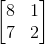Explanation:

To find the determinant of a 2x2 matrix, we use the formulaApplying to the matrix from the problem statement, we get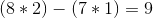Example Question #748 : Vectors And Vector Operations

Find the determinant of the 3x3 matrix given: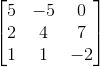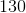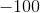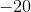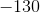Explanation:

To find the determinant of a 3x3 matrix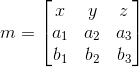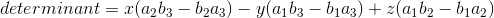Using the vectors from the problem statement, we get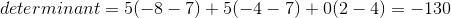Example Question #749 : Vectors And Vector Operations

Find the determinant of the 3x3 matrix given: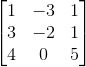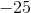Explanation:

To find the determinant of a 3x3 matrixUsing the vectors from the problem statement, we get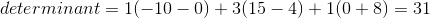Example Question #750 : Vectors And Vector Operations

Find the determinant of the 3x3 matrix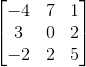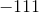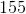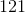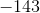Explanation:

To find the determinant of a 3x3 matrix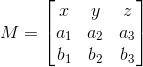, you use the formula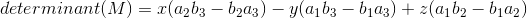Using the matrix from the problem statement, we get